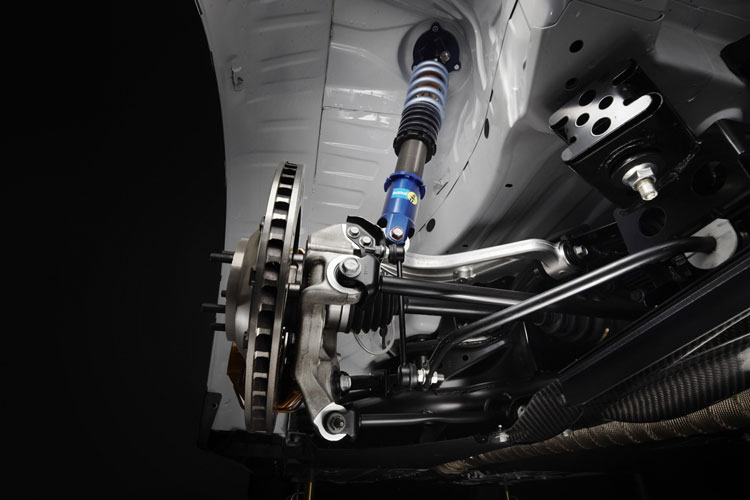What is an Installation Ratio?

An installation ratio is the ratio between how much the spring and damper moves in relation to how far the wheel and tyre move. In general, the wheel and tyre will move a further distance than the spring and damper. The installation ratio is vital to understand in your suspension set up particularly if you are planning on choosing you own spring rates as it helps to determine the effective spring rate at the wheel known as “wheel rate”. It therefore determines the amount of deflection that the spring and damper and anti-roll bar will experience at any displacement of the wheel and tyre.

The installation ratio for the spring/damper and the installation ratio for the anti-roll bar will both need measuring or calculating for the front and rear wheels. The values will then allow the correct spring rates to be installed on the car or will help to understand the actual spring rate experienced at the wheel (known as wheel rate) on your current set up.

What Equipment Do I Need?Calculating the installation ratio is a simple process and requires little equipment. The equipment required to be able to carry out both of the following techniques are:

• Tape Measure
• Axle Stands
• Hydraulic Trolley Jack
• Spanners to Remove Dampers and Anti-Roll Bar Drop Links

How to Measure or Calculate the Installation Ratio

The equation used to calculate the installation ratio is very simple and is shown below:There are two techniques that can be used to determine installation ratio. One is to take a series of physical measurements from the car. The other is to measure the pick-up point of the damper and the length of the lower arm to calculate it. In the case where the car uses a bell crank in such set ups as push or pull rod suspension, or in scenarios where a MacPherson strut set up is used, this series of measurements is the best route to take. If you have a conventional set up in the case of many road cars such as double wish bone or lower arm mounted dampers, the second technique might be a faster process for you.Technique 1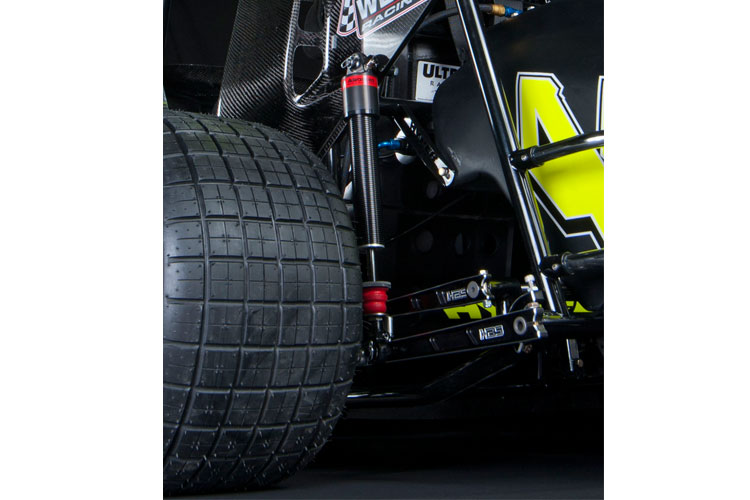For the first technique of taking a series of measurements, it is best to measure the length of the damper when the car is at ride height first. Then, raise the car off the ground and sit it on axle stands at a height where a jack can be inserted below the wheel. If it is then possible it is easier if the coil spring can be removed from the damper (like in the image above) so that the car is not lifted off the axle stands when the wheel has been compressed by the jack. It is also possible to carry out this task with the damper entirely removed. Finally disconnect the anti-roll bar by unbolting the drop link from the system.

With the wheel sat in the same position it was at ride height, measure how far from the floor the centre of the wheel is. Now measure how far the upper and lower damper mounting positions are and make a note of both of these figures. The table below can be used for measurements.

 Wheel Displacement (mm) Damper Displacement (mm) Installation Ratio -50 -45 -40 -35 -30 -25 -20 -15 -10 -5 0 0 0 5 10 15 20 25 30 35 40 45 50

Now move the jack up so it is 5mm higher than ride height and measure the distance between the upper and lower damper mounting points. Next, put the difference between the first measurement and the compressed measurement in the table above. Then repeat the process, raising the wheel by 5mm each time and using the below equation to populate the table.With these measurements taken return the jack to ride height and take the same measurements in the same 5mm gaps but in the reverse direction for droop or rebound movement of the wheel. With the first two columns now full; use the below equation to calculate the installation ratio to fill the final column. The equation is:The value of the installation ratio might alter for each row. This is fine as some suspension systems alter due to the arc pattern that the suspension takes when being moved up and down in bump and droop.

If your readings are all similar for the installation ratio then you can calculate the installation ratio for your car by simply adding together all of the values you have written down for installation ratio in the table and then divide that number by the amount of measurements you have taken. Ignore the ride height row for this calculation as the 0 value is irrelevant.

However, if your values for installation ratio alter by a noticeable amount then it would be best to put these values into excel to generate a graph similar to below to generate your installation ratio at any given point in the suspension range. This can be used when speccing spring rates for a certain optimum operating range.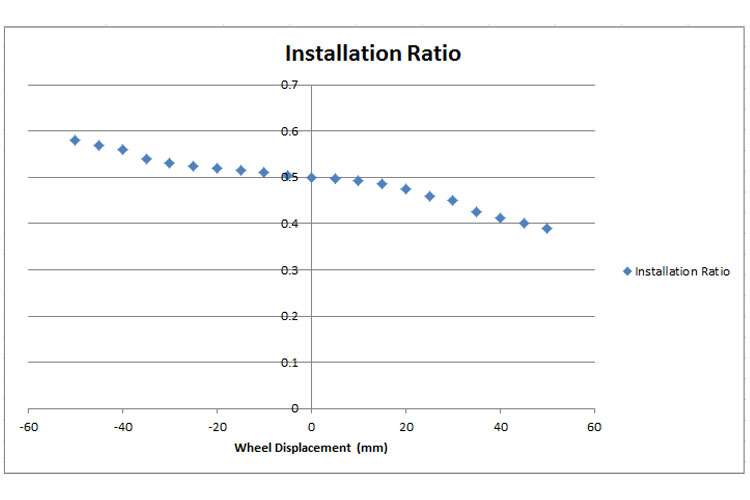Technique 2

If your car has the damper mounted to the lower arm at the front or the rear then it can be faster to simply take measurements of the pick-up points and use the equation further down to calculate the installation ratio. The diagram below shows the two measurements that need to be taken to calculate the installation ratio of the wheel.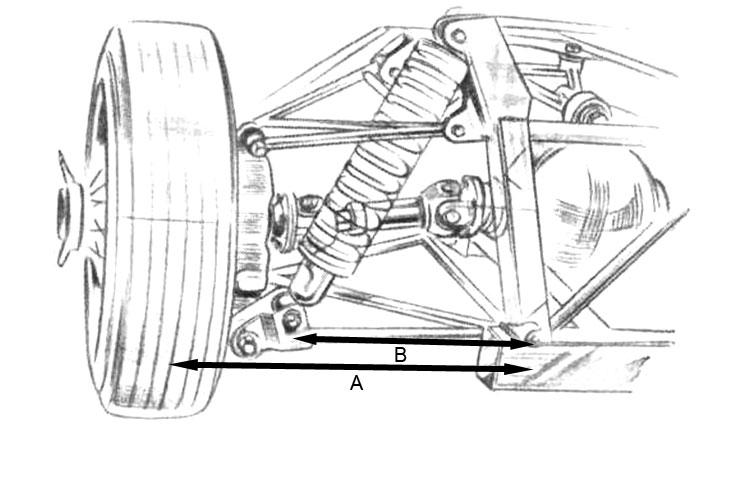As seen in the image above we can measure the length of the lower arm or from the subframe mounting bolt to the centre point of the wheel and the distance from the in board mounting point where the arm mounts to the chassis or subframe to where the damper mounts on the arm.

For a MacPherson strut set up it is still possible to measure the points to calculate the installation ratio. For this set up you need to measure down from the lower arm mounting bolt directly to the centre of the contact patch of the tyre. Then draw a line perpendicular to the damper until it meets that line. These two lengths become length A and Length B as shown in the image below.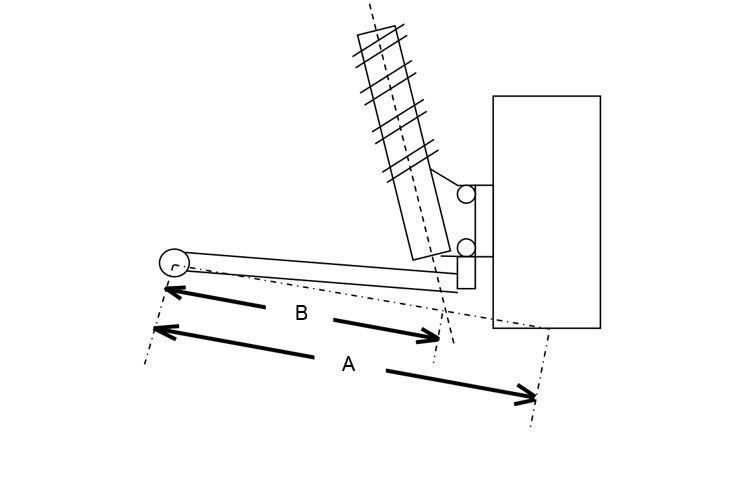Now with these two measurements simply input them into the equation below to produce the installation ratio of your set up.Anti-Roll Bar Installation Ratio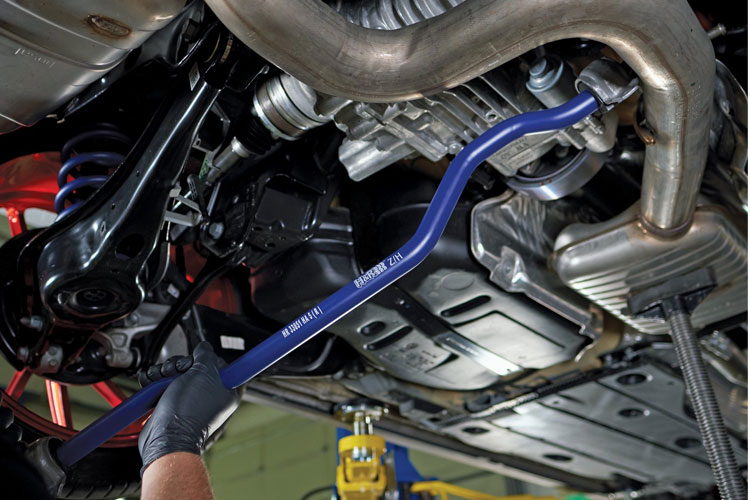In the frequent case where the anti-roll bar is mounted to the lower arm via a drop link, technique 2 can be used in exactly the same way but distance 2 is a measurement from the chassis or subframe mounting point for the arm to where the drop link mounts to the arm. These measurements can be used in the above equation in the same way to calculate the anti-roll bar installation ratio.If the roll bar is mounted using a bell crank like in many push or pull rod suspension systems, then technique 1 can be used with the table being printed off. However, for this the anti-roll bar must be disconnected from the bell crank to allow free movement of the wheel. The table can then be populated with how much the bell crank moves towards the anti-roll bar (distance B in the above image). Then using the same equations as for the damper measurements the anti-roll bar installation ratio will be produced.Motion Ratio

The motion ratio of the suspension is simply a function of the installation ratio and is a theoretical value as oppose to a physical measurement. The motion ratio is required for calculating the effective spring rate at the wheel known as the wheel rate which is a fundamental figure for understanding vehicle dynamics and calculating ideal spring rates.  The motion ratio can be calculated very easily once the installation ratio has been calculated. The equation for the motion ratio is shown below:OrThis value can be noted down and used for wheel rate calculations.